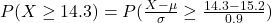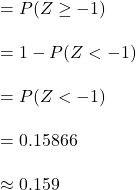## at one high school, the mean time for running the 100-yard dash is 15.2 seconds with a standard deviation of 0.9 seconds. the times are very

Question

at one high school, the mean time for running the 100-yard dash is 15.2 seconds with a standard deviation of 0.9 seconds. the times are very closely approximated by a normal curve. find the percent of times that are at least 14.3 seconds

in progress 0
2 weeks 2021-08-28T18:35:38+00:00 1 Answers 0 views 0

The percent of times that are at least 14.3 seconds is approximately 16%.

Step-by-step explanation:

Let X denote the time for running the 100-yard dash.

It is provided that the times are very closely approximated by a normal curve.

So, the random variable X follows a normal distribution with a mean time of 15.2 seconds and with a standard deviation of 0.9 seconds.

Compute the probability of times that are at least 14.3 seconds as follows:*Use a z-table.

Thus, the percent of times that are at least 14.3 seconds is approximately 16%.